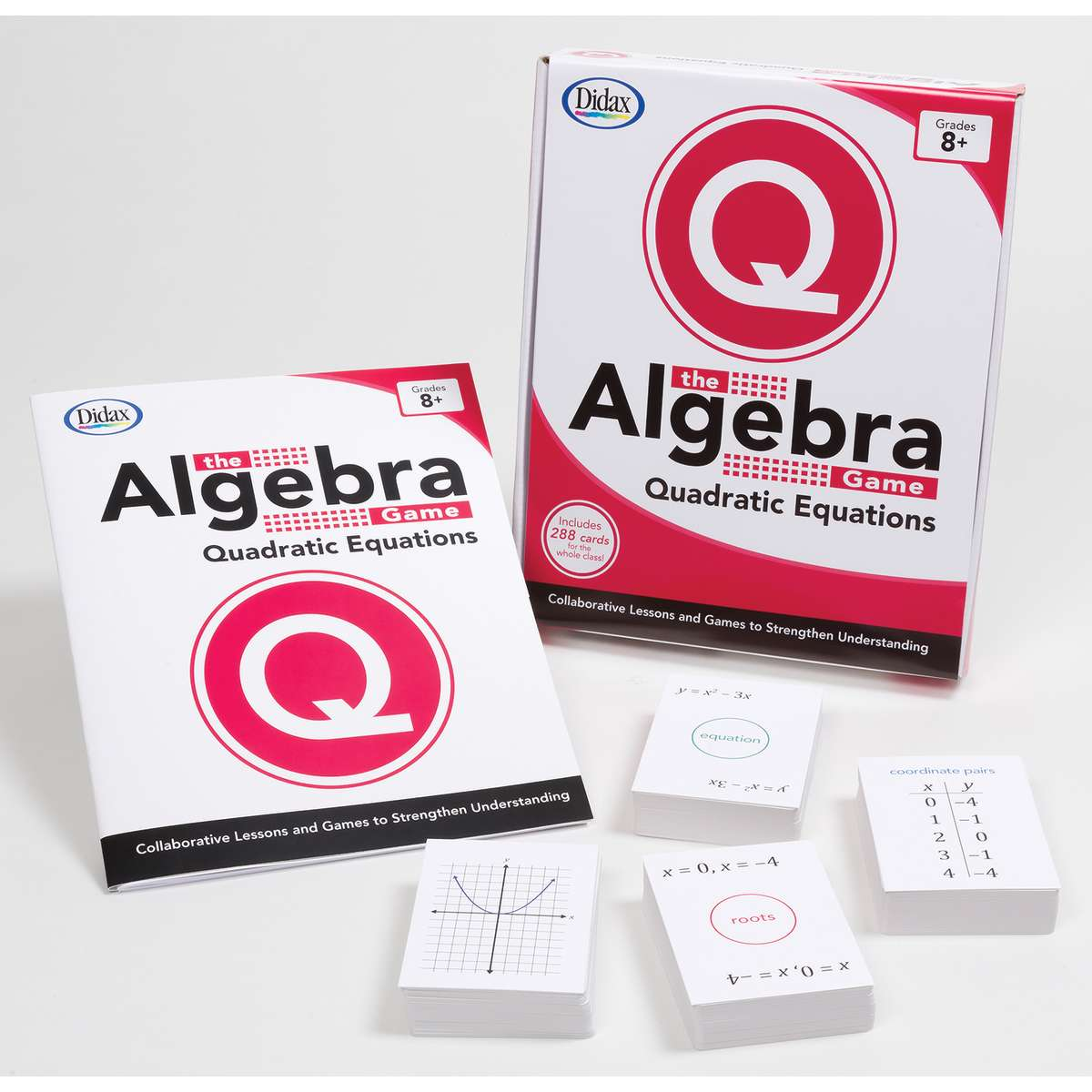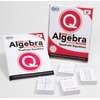•# The Algebra Game, Quadratic Equations: Basic

Product Number: DD-211754
\$52.99
Up to 12 students can work collaboratively to form a multitude of algebraic equations in each area of study. Each set include...
Quantity

Up to 12 students can work collaboratively to form a multitude of algebraic equations in each area of study. Each set includes 4 decks of cards and a comprehensive teacher’s guide featuring lessons, games, discussion questions, and reproducible activities.
DD-211754